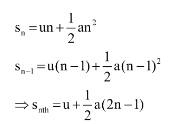#### Obtain a relation for the distance travelled by an object moving with uniform acceleration in the interval between 4th and 5th seconds.Distance travelled in nth second by any particle is equal to the difference of distances travelled in n seconds and n -1 seconds.

For example. distance travelled in the fourth second is the difference of distance travel in four seconds and three seconds.

Hence, by using the second equation of motion we can find out the distance travelled in nth second.If the initial velocity is zero,

In that case, the distance travelled in the fourth second and fifth second will be in the ratio of 7:9.